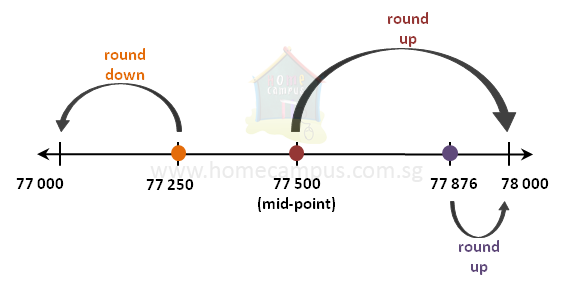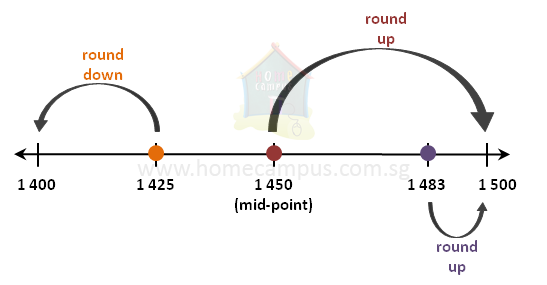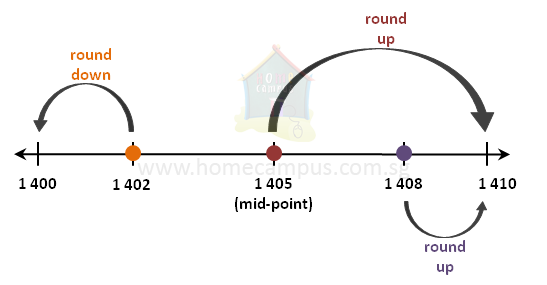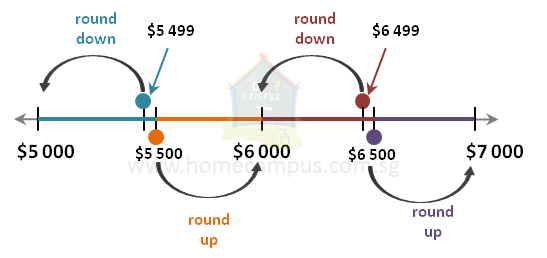## Approximation and Estimation Part 1

Practice Unlimited Questions

#### What is approximation?

Approximation means rounding off. We round off numbers to simplify them so they are easy to use and easy to remember.

Examples:
1. You bought a pair of shoes for \$99. If someone asks you the price of the shoes you are more likely to say that the price is \$100.
1. This is called rounding up where the new number is bigger than the given number.
2. \$99 ≈ \$100 (You say it as: \$99 is approximately equal to \$100)
2. A dress costs \$81. You will more likely to say that the cost of the dress was \$80.
1. This is called rounding down where the new number is smaller than the given number.
2. \$81 ≈ \$80 (You say it as: \$81 is approximately equal to \$80)

#### 1.  a)   Round off 77 250 to the nearest 1000 b)   Round off 77 500 to the nearest 1000 c)   Round off 77 876 to the nearest 1000

Rounding off to the nearest thousand

When rounding off to the nearest 1000, you only care about the part of the number up to the thousands place. So,
1. Check the digit in the hundreds place.
1. If it is less than 5, keep the digit in the thousands place unchanged (i.e. round down)
2. If it is equal to or more than 5, add 1 to the digit in the thousands place (i.e. round up)
2. Change the digits in the hundreds place, tens place and ones place to 0s.1. 77 250 ≈ 77 000 (when rounded off to the nearest 1000, it is rounded down because it is closer to 77 000 than to 78 000)
2. 77 500 ≈ 78 000 (when rounded off to the nearest 1000, it is rounded up because it is closer to 78 000 than to 77 000)
3. 77 876 ≈ 78 000 (when rounded off to the nearest 1000, it is rounded up because it is closer to 78 000 than to 77 000)

Notes:
1. All numbers to the left of the midpoint on the number line will be rounded down.
2. All numbers to the right of the midpoint including the midpoint itself will be rounded up.

#### 2. a)   Round off 1425 to the nearest 100 b)   Round off 1450 to the nearest 100 c)   Round off 1483 to the nearest 100

Rounding off to the nearest hundred

When rounding off to the nearest 100, you only care about the part of the number up to the hundreds place. So,
1. Check the digit in the tens place.
1. If it is less than 5, keep the digit in the hundreds place unchanged (i.e. round down)
2. If it is equal to or more than 5, add 1 to the digit in the hundreds place (i.e. round up)
2. Change the digits in the tens place and ones place to 0s.1. 1425 ≈ 1400 (when rounded off to the nearest 100, it is rounded down because it is closer to 1400 than to 1500)
2. 1450 ≈ 1500 (when rounded off to the nearest 100, it is rounded up because it is closer to 1500 than to 1400)
3. 1483 ≈ 1500 (when rounded off to the nearest 100, it is rounded up because it is closer to 1500 than to 1400)

#### 3. a)   Round off 1402 to the nearest 10 b)   Round off 1405 to the nearest 10 c)   Round off 1408 to the nearest 10

Rounding off to the nearest ten

When rounding off to the nearest 10, you only care about the part of the number up to the tens place. So,
1. Check the digit in the ones place.
1. If it is less than 5, keep the digit in the tens place unchanged (i.e. round down)
2. If it is equal to or more than 5, add 1 to the digit in the tens place (i.e. round up)
2. Change the digits in the ones place to 0.1. 1402 ≈ 1400 (when rounded off to the nearest 10, it is rounded down because it is closer to 1400 than to 1410)
2. 1405 ≈ 1410 (when rounded off to the nearest 10, it is rounded up because it is closer to 1410 than to 1400)
3. 1408 ≈ 1410 (when rounded off to the nearest 10, it is rounded up because it is closer to 1410 than to 1400)

#### 4. If Jack has an income of \$6000 when rounded off to the nearest thousand. What could his actual income be?1. When a number given to you has been round off to the nearest thousand, then there are 1000 possible options that the actual number could be.
1. Half of those numbers (500 of them) would be less than the given number, and
2. Half of those numbers (500 of them) would be equal to or greater than the given number.
2. When rounding off to the nearest 1000, all numbers from \$5500 to \$5999 will be rounded up to give \$6000.
3. When rounding off to the nearest 1000, all numbers from \$6001 to \$6499 will be rounded down to give \$6000.

So, Jack's actual income could be anywhere between \$5500 and \$6499.

#### 5. If Jack has an income of \$5920 and Harry has an income of \$4120, estimate the sum of money they earn together.

Jack's income  =  \$5920  ≈  \$6000

Harry's income  =  \$4120  ≈  \$4000

Total  =  Jack's income  +  Harry's income
≈  \$6000  +  \$4000
=  \$10 000

Jack and Harry earn approximately \$10 000 together.